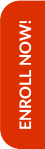Olympiads are the stepping stones to achieve better results in the competitive world that lies ahead in the life of the child. Math Olympiad examinations help students to improve their mathematical skills along with their analytical and problem solving abilities. Along with that CBSE syllabus also plays a crucial role in the same.

Hence, Olympiad Success Live has designed a long-term course for Mathematics preparation which includes both CBSE syllabus and Olympiad for class 7. This long-term course will help in building the foundation of the child. For this, we have done great efforts in finding the tutor for class 7 Math CBSE and Olympiad preparation with relevant background and experience.

If you are interested in purchasing this course, then please Enrol Now. You will be redirected to the batch detail page, wherein you can see all the details like batch start and demo dates, fess and the registration link related to Math CBSE and Olympiad for class 7 course.

Grade 7 CBSE Mathematics syllabus identifies age-appropriate content. During the learning of Mathematics from grade 6 to grade 8, a child:

• Moves from number sense to number patterns;
• Sees relationships between numbers and looks for patterns in relationships;
• Gains proficiency in using the newer language of Mathematics like variables, expressions, equations, identities, etc.
• Uses arithmetic and algebra to solve real-life problems and pose meaning problems;
• Discovers symmetries and acquire a sense of aesthetics by looking around regular shapes like triangles, circles, quadrilaterals, etc;
• Comprehends the idea of space as reason enclosed within boundaries of a shape;
• Relates numbers with shapes in terms of perimeter, area and volume and uses them to solve everyday life problems;
• Learns to provide reasoning and convincing arguments to justify her/his own conclusions particularly in Mathematics; and
• Collects, represents (graphically and in tables) and interprets data/information from her/his life experiences

The content of grade 7 CBSE Maths is consistent with the development of the student which includes:

• Numbers
• Algebraic Expressions
• Ratio and Proportion
• Geometry - Understanding shapes
• Geometry - Properties of triangles
• Geometry - Symmetry
• Representing 3-D in 2-D
• Congruence
• Construction
• Mensuration
• Area
• Data Handling

Check the detailed syllabus below. For Olympiads preparation, you can check the syllabus here!

Keeping in mind the curricular expectations, Olympiad Success Live has designed a course for CBSE and Olympiads Maths preparation for class 7 students. And for this, we have the right set of tutors with relevant backgrounds and experience for class 7 Maths CBSE and Olympiads preparation.

If you are interested in enrolling in this grade 7 CBSE and Olympiad Math course, then you can pay the fees here. After payment, kindly WhatsApp us your name, class and subject at +91 95607 64447

##### Syllabus

Numbers

• Multiplication and division of integers
• Properties of integers (including identities for addition and multiplication, commutative, associative, distributive)
• Word problems including integers
• Operations on integers
• Multiplication and division of fractions
• Mixed fractions
• Rational numbers
• Operations on rational numbers
• Decimal representation of rational numbers
• Multiplication and division of decimal fractions
• Units conversion of length and mass from smaller to larger and vice-versa
• Operations on rational numbers and decimal fractions
• Exponents and their laws

Algebraic Expressions

• Constants, coefficient, powers, like and unlike terms and degree of an expression
• Addition and subtraction of algebraic expressions
• Simple linear equations in one variable (in contextual problems) with two operations

Ratio and Proportion

• Ratio and proportion and Unitary method
• Percentage as a fraction with denominator
• Fractions and decimals into percentage and vice-versa
• Profit and loss (single transaction only)
• Simple interest (time period in complete years)

Geometry - Understanding shapes

• Pairs of angles (linear, supplementary, complementary, adjacent, vertically opposite)
• Properties of parallel lines with transversal (alternate, corresponding, interior, exterior angles)

Geometry - Properties of triangles

• Angle sum property and exterior angle property of a triangle
• Sum of two sides of a triangle is greater than the third side of a triangle
• Pythagoras Theorem (Verification only)

Geometry - Symmetry

• Reflection symmetry
• Rotational symmetry, observations of rotational symmetry of 2-D objects

Representing 3-D in 2-D

• Vertices, edges, faces and nets (for cubes cuboids, and cylinders, cones)
• Maps of the space

Congruence

• Congruence through superposition
• Congruence to simple geometrical shapes e.g. triangles, circles
• Criteria of congruence (SSS, SAS, ASA, RHS)

Construction

• Parallel lines
• Simple triangles

Mensuration

• Perimeter and idea of the circumference of a circle
• Pie

Area

• Concept of measurement - square, rectangle, triangle, parallelogram and circle

Data Handling

• Collect and organise data
• Mean, median and mode of ungrouped data
• Bar
• Probability

Online classes started for English, Mathematics, Science and Reasoning for classes (3rd to 10th)